# Riverboat Problems

Example: Boat has velocity 5m/s with respect to the river. It aims to reach point A, however, because of the river speed it reaches another point B. If the speed of current is 2m/s to the east calculate the time of trip and the distance between A and B.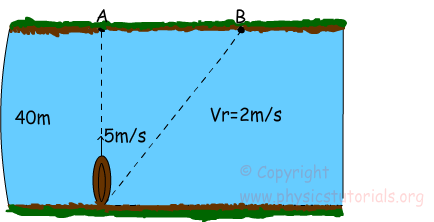Boat follows the path shown in dashed line which is the direction of the resultant vector.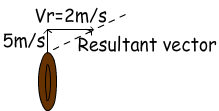This example can be examined under two part vertical and horizontal motion as in the case of projectile motion.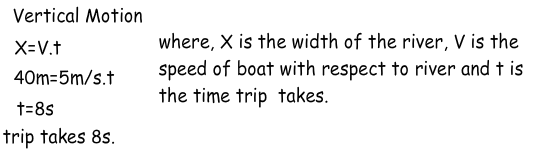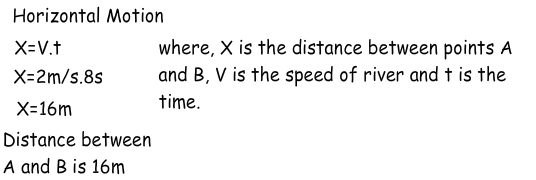Example: Velocity of the boat with respect to river is 10 m/s. It passes the river and reaches opposite shore at point C. If the velocity of the river is 3m/s, find the time of the trip and distance between B and C.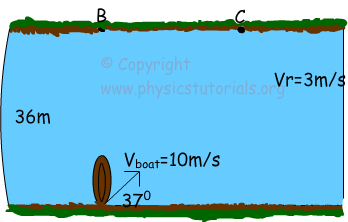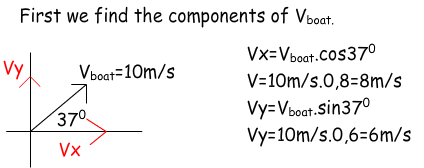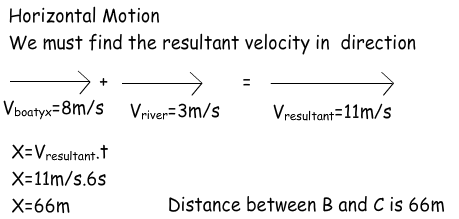Kinematics Exams and Solutions